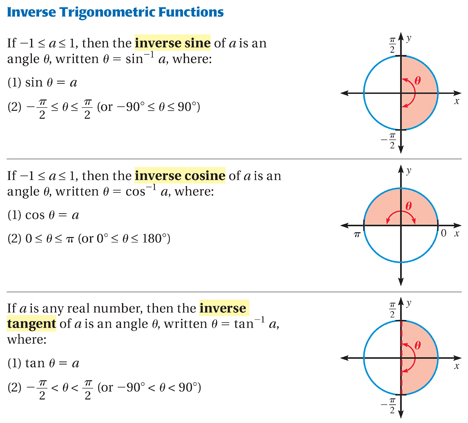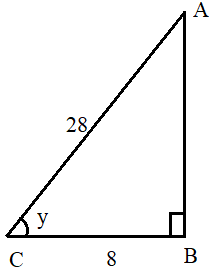#### Arccos Calculator

Result:

 Angle in degrees: 0 Angle in radian: 0

### What is arccos?

Arccosine (or arccos) is the inverse of the cosine function. In a right-angled triangle, the cos of an angle y is defined as the ratio of the side adjacent to the angle, to the hypotenuse. When we take arccosine of this ratio, we get the measure of the angle y.

Consider the right-angled triangle below.In this triangle, the right angle is at B. Cos of the angle y is given by

$$cos\, y\, =\, {adjacent \over hypotenuse} \,=\,{BC\over AC} = x$$

To find the angle y when the ratio BC/AC is known, we use the following formula for arccosine.

$$y\, =\, cos^{-1}{adjacent \over hypotenuse} \,= cos^{-1}\,{BC \over AC}\, = cos^{-1} x$$

The expression y = cos-1⁡x, means that cos y = x , when 0 ≤ y ≤ π and -1≤ x ≤ +1.

Arccosine(x)=y is defined as the set of all angles whose cosine is x. It should be noted here that the -1 is not an exponent and hence, cos-1⁡x ≠ 1 / cos x .

### Properties of the arccosine function

Given below are some properties of the arccosine function.

Angle – The angle is the parameter which is calculated when cos inverse (or arccosine) of the angle is known.

Domain – The arccosine of the parameter whose cos is x. This value goes from -1 ≤ x ≤ 1.

Range – The angles for which we can find cos^(-1)⁡x. This value is 0 ≤ y ≤ π radians or 0 ≤ y ≤ +180 degrees.

Given below is a graph of the arccosine function.In the above graph, the y-axis denotes the angle in radians, and the x-axis denotes arccosine of that angle. The red curve denotes the arccosine function. For any value of x between -1 and +1, the arccosine function returns a value between 0 and π radians (or 0⁰ to 180⁰). For example, when x=1, y=arccosine(x) returns y= 0 radians. This means that cos (0) = 1.

If the arccosine graph is compared to the sine graph, one would see that the arccosine graph can be obtained from the cosine graph by simply interchanging the horizontal and vertical axes.

### Values of arcsine(x)

Given below are some commonly used values of arccosine(x).

y=cos-1⁡x in degrees y=cos-1⁡x in radians x
0 0 1
30 $$π\over6$$ $$√3\over2$$
45 $$π\over4$$ $$1\over√2$$
60 $$π\over3$$ $$1\over2$$
90 $$π\over2$$ 0
120 $$2π\over3$$ $$-1\over2$$
135 $$3π\over4$$ $$-1\over√2$$
150 $$5π\over6$$ $$-√3\over2$$
180 $$π$$ -1

The value of arccosine(x) is always positive, even when x is negative. For example, arccosine(-1/2) = 120⁰ and arccosine(1/2) = 60⁰ are both positive. This is because cos-1⁡(-x)=-cos-1⁡x.

This has been illustrated below.

In this figure, the x-axis denotes the arccosine values, whereas the y-axis denotes the angle θ. Arccosine(x) will be positive when 0 ≤ θ ≤ π/2 (in Quadrant 1), and negative when π/2 < θ ≤ π. (in Quadrant 2).We can also note that

cos⁡(cos-1⁡(x))=x when -1 ≤ x ≤ +1.

For example, cos⁡(cos-1⁡(1/2)) = cos⁡ 60⁰ = 1/2.

Similarly, cos-1⁡⁡(cos⁡ x) = x when 0 ≤ x ≤ +π.

For example, cos-1⁡⁡(cos⁡ π/4) = cos-1⁡⁡⁡(1/√2) = π/4.

If we need to find the arccosine of the cos of an angle which does not lie between 0 and π, the correct angle can be found by either adding or subtracting 2π radians until we get an angle in the range of 0 to π, which is the range of the arccosine function. This is because 2π is the period of the cos function.

### Arccosine formula

From a right-angled triangle, the arccosine formula can be computed as

$$y = cos^{-1}{BC \over AC} = cos^{-1} \,x$$

where BC is the adjacent side and AC is the hypotenuse, and y is the angle which can be calculated.

Like cos(x), there is no well-known Taylor series approximation for the arccosine function. However, a series expansion can be obtained if the expansion for the arcsine function is known, using the following formula.

$$cos^{-1}x = {π\over2} - sin^{-1} x$$

The formula thus obtained, where -1 < x < 1, is given below.

$$cos^{-1}x = {π\over2} - (x + {1\over2}\,{x^3\over3} + {1.3\over2^2\,2!}{x^5\over5} + {1.3.5\over2^3 3!}{x^7\over7} + {1.3.5.7\over2^4 4!}{x^9\over9} + ...)$$

### Areas of application

Trigonometric functions as well as the inverse trigonometric functions are used widely in engineering, geometry and physics. In everyday life, indirect measurement is used to find answers for problems which cannot be solved easily using measurement tools. In such cases, trigonometric and inverse trigonometric functions find extensive use to calculate these unknown measurements.

They are also used to solve trigonometric equations describing real-life problems.

In this section, we consider some example problems involving the use of the arccosine formula.

Question: Compute cos-1⁡(cos -6π/5).

Answer:The angle -6π/5 radians does not lie between 0 and π, but it can be brought to this range by either adding or subtracting 2π radians until we get an angle in the range of 0 to π radians, which is the range of the arccosine function.

In this case, -6π/5 radians can be written as -6π/5+2π=4π/5 radians, which lies between 0 and π.

Therefore, cos-1⁡(cos⁡ -6π/5) = cos-1⁡ (cos⁡(4π/5) ) = 4π/5

Question: A man walks in the northeast direction for 28 miles, and he realizes that he is 8 miles away from his starting point. In what direction was he walking?

Answer: The problem can be visualized as below.In the given right-angled triangle, the sides are as follows. We need to find the angle y.

AC indicates the distance covered by the man in the northeast direction, and BC is the distance from his starting point. This can be visualized as a right-angled triangle as shown above. Here, AC= 28 miles, and BC= 8 miles.

To find the direction in which he was walking, we can use the cosine function.

$$cos\, y = {adjacent\over hypotenuse} = {8\over28} = {2\over7}$$

$$cos\, y = {2\over7}$$

$$y = cos^{-1}({2\over7}) ≈ 73.398⁰$$

Hence, the man was walking 73.4⁰ north of east.